# Flink 1.9 Table API& Sql支持的Data Type

Flink 1.9 Table API & SQL 新特性主要包括如下：

• 全新的 SQL 类型系统：Table API & SQL 1.9 引入了全新的 SQL 的类型系统。以往的Table 层的类型系统复用了 Runtime 的 TypeInformation，但在实际操作过程当中遇到较多的限制。引入全新的 SQL 类型系统可以更好的对齐 SQL 语义。
• DDL初步支持：这个版本中 Flink 还引入了 DDL 的初步支持，用户可以使用 Create Table 或 Drop Table 等简单的语法定义表格或删除表。
• Table API增强：Table API 原来仅为关系表达式的 API，Table API & SQL 1.9中现在加入了 Map，FlatMap 等更加灵活的 API。
• 统一的Catalog API：Table API & SQL 1.9 引入了统一的 Catalog API 之后，可以方便的和其它的 Catalog 对接。比如常见的 Hive，可以通过统一的 Catalog API，实现与 Hive.metastore 交互的插件，让 Flink 可以直接读取和处理 Hive 中的表。
• Blink planner：Table API 增加了 Blink planner 的支持，因为在底层的 Runtime 做了较大的变化后，上层需要 SQL 的 Planner 与底层的 Runtime 进行对接。为了确保原来的 Table API 用户尽量不受影响，社区完整保留了原来的 Flink Planner。但同时又引入了新的 Blink planner，与新的 Runtime 设计进行对接。

**注意：**在使用数据类型之前，请参阅执行计划（planner）兼容性Table和限制部分。

## 数据类型

• `INT`
• `INT NOT NULL`
• `INTERVAL DAY TO SECOND(3)`
• `ROW<myField ARRAY<BOOLEAN>, myOtherField TIMESTAMP(3)>`

## Table API中的数据类型

DataType实例有两个职责：

**逻辑类型的声明：**这并不意味着传输或存储的具体物理表示，而是定义了基于JVM的语言和表生态系统之间的界限。

**可选的：**向执行计划提供有关数据物理表示的提示，这对于其他API的边缘很有用。

``````import org.apache.flink.table.api.DataTypes._

val t: DataType = INTERVAL(DAY(), SECOND(3));
``````

### 物理提示

``````// tell the runtime to not produce or consume java.time.LocalDateTime instances
// but java.sql.Timestamp
val t: DataType = DataTypes.TIMESTAMP(3).bridgedTo(classOf[java.sql.Timestamp]);

// tell the runtime to not produce or consume boxed integer arrays
// but primitive int arrays
val t: DataType = DataTypes.ARRAY(DataTypes.INT().notNull()).bridgedTo(classOf[Array[Int]]);
``````

**注意：**通常只有在扩展API时才需要物理提示。sources/sinks/functions的无需定义此类提示。Table程序中的提示（例如field.cast(TIMESTAMP(3).bridgedTo(Timestamp.class))）将被忽略。

## 执行计划（Planner）兼容性

### Old Planner

Type Information Java Expression String Data Type Representation
`STRING()` `STRING` `STRING()`
`BOOLEAN()` `BOOLEAN` `BOOLEAN()`
`BYTE()` `BYTE` `TINYINT()`
`SHORT()` `SHORT` `SMALLINT()`
`INT()` `INT` `INT()`
`LONG()` `LONG` `BIGINT()`
`FLOAT()` `FLOAT` `FLOAT()`
`DOUBLE()` `DOUBLE` `DOUBLE()`
`ROW(...)` `ROW<...>` `ROW(...)`
`BIG_DEC()` `DECIMAL` `[DECIMAL()]`
`SQL_DATE()` `SQL_DATE` `DATE().bridgedTo(java.sql.Date.class)`
`SQL_TIME()` `SQL_TIME` `TIME(0).bridgedTo(java.sql.Time.class)`
`SQL_TIMESTAMP()` `SQL_TIMESTAMP` `TIMESTAMP(3).bridgedTo(java.sql.Timestamp.class)`
`INTERVAL_MONTHS()` `INTERVAL_MONTHS` `INTERVAL(MONTH()).bridgedTo(Integer.class)`
`INTERVAL_MILLIS()` `INTERVAL_MILLIS` `INTERVAL(DataTypes.SECOND(3)).bridgedTo(Long.class)`
`PRIMITIVE_ARRAY(...)` `PRIMITIVE_ARRAY<...>` `ARRAY(DATATYPE.notNull().bridgedTo(PRIMITIVE.class))`
`PRIMITIVE_ARRAY(BYTE())` `PRIMITIVE_ARRAY<BYTE>` `BYTES()`
`OBJECT_ARRAY(...)` `OBJECT_ARRAY<...>` `ARRAY(DATATYPE.bridgedTo(OBJECT.class))`
`MULTISET(...)` `MULTISET(...)`
`MAP(..., ...)` `MAP<...,...>` `MAP(...)`
other generic types `ANY(...)`

Data Type Remarks for Data Type
`STRING` `CHAR` and `VARCHAR` are not supported yet.
`BOOLEAN`
`BYTES` `BINARY` and `VARBINARY` are not supported yet.
`DECIMAL` Supports fixed precision and scale.
`TINYINT`
`SMALLINT`
`INTEGER`
`BIGINT`
`FLOAT`
`DOUBLE`
`DATE`
`TIME` Supports only a precision of `0`.
`TIMESTAMP` Supports only a precision of `3`.
`TIMESTAMP WITH LOCAL TIME ZONE` Supports only a precision of `3`.
`INTERVAL` Supports only interval of `MONTH` and `SECOND(3)`.
`ARRAY`
`MULTISET`
`MAP`
`ROW`
`ANY`

## 局限性

Java Expression String: Table API中的Java表达式字符串，例如table.select（“ field.cast（STRING）”）尚未更新为新类型的系统。使用old planner部分中声明的字符串表示形式。

Connector Descriptors and SQL Client:描述符字符串表示形式尚未更新为新类型系统。使用在“Connect to External Systems section”部分中声明的字符串表示形式

User-defined Functions: 用户定义的函数尚不能声明数据类型。

## Data Type 列表

### 字符串

#### `CHAR`

SQL

``````CHAR
CHAR(n)
``````

JAVA/SCALA

``````DataTypes.CHAR(n)
``````

STRING是VARCHAR（2147483647）的同义词。

#### `VARCHAR` / `STRING`

SQl

``````VARCHAR
VARCHAR(n)

STRING
``````

JAVA/SCALA

``````DataTypes.VARCHAR(n)

DataTypes.STRING()
``````

STRING是VARCHAR(214748364)的同义词。

Java Type Input Output Remarks
`java.lang.String` X X Default
`byte[]` X X Assumes UTF-8 encoding.

### 二进制字符串

#### `BINARY`

SQL

``````BINARY
BINARY(n)
``````

JAVA/SCALA

``````DataTypes.BINARY(n)
``````

Java Type Input Output Remarks
`byte[]` X X Default

### 精确数值

#### `DECIMAL`

SQL

``````DECIMAL
DECIMAL(p)
DECIMAL(p, s)

DEC
DEC(p)
DEC(p, s)

NUMERIC
NUMERIC(p)
NUMERIC(p, s)
``````

JAVA/SCALA

``````DataTypes.DECIMAL(p, s)
``````

NUMERIC（p，s）和DEC（p，s）是此类型的同义词。

Java Type Input Output Remarks
`java.math.BigDecimal` X X Default

#### `TINYINT`

1字节有符号整数的数据类型，其值从-128到127。

SQL

``````TINYINT
``````

JAVA/SCALA

``````DataTypes.TINYINT()
``````

Java Type Input Output Remarks
`java.lang.Byte` X X Default
`byte` X (X) Output only if type is not nullable.

#### `SMALLINT`

2字节有符号整数的数据类型，其值从-32,768到32,767。

SQL

``````SMALLINT
``````

JAVA/SCALA

``````DataTypes.SMALLINT()
``````

Java Type Input Output Remarks
`java.lang.Short` X X Default
`short` X (X) Output only if type is not nullable.

#### `INT`

SQL

``````INT

INTEGER
``````

JAVA/SCALA

``````DataTypes.INT()
``````

INTEGER是此类型的同义词。

Java Type Input Output Remarks
`java.lang.Integer` X X Default
`int` X (X) Output only if type is not nullable.

#### `BIGINT`

SQL

``````BIGINT
``````

JAVA/SCALA

``````DataTypes.BIGINT()
``````

Java Type Input Output Remarks
`java.lang.Long` X X Default
`long` X (X) Output only if type is not nullable.

### 近似数值

#### `FLOAT`

4字节单精度浮点数的数据类型。与SQL标准相比，该类型不带参数。

SQL

``````FLOAT
``````

JAVA/SCALA

``````DataTypes.FLOAT()
``````

Java Type Input Output Remarks
`java.lang.Float` X X Default
`float` X (X) Output only if type is not nullable.

#### `DOUBLE`

8字节双精度浮点数的数据类型。

SQL

``````DOUBLE

DOUBLE PRECISION
``````

JAVA/SCALA

``````DataTypes.DOUBLE()
``````

DOUBLE PRECISION是此类型的同义词。

Java Type Input Output Remarks
`java.lang.Double` X X Default
`double` X (X) Output only if type is not nullable.

### 日期和时间

#### `DATE`

SQL

``````DATE
``````

JAVA/SCALA

``````DataTypes.DATE()
``````

Java Type Input Output Remarks
`java.time.LocalDate` X X Default
`java.sql.Date` X X
`java.lang.Integer` X X Describes the number of days since epoch.
`int` X (X) Describes the number of days since epoch. Output only if type is not nullable.

#### `TIME`

SQL

``````TIME
TIME(p)
``````

JAVA/SCALA

``````DataTypes.TIME(p)
``````

Java Type Input Output Remarks
`java.time.LocalTime` X X Default
`java.sql.Time` X X
`java.lang.Integer` X X Describes the number of milliseconds of the day.
`int` X (X) Describes the number of milliseconds of the day. Output only if type is not nullable.
`java.lang.Long` X X Describes the number of nanoseconds of the day.
`long` X (X) Describes the number of nanoseconds of the day. Output only if type is not nullable.

#### `TIMESTAMP`

SQL

``````TIMESTAMP
TIMESTAMP(p)

TIMESTAMP WITHOUT TIME ZONE
TIMESTAMP(p) WITHOUT TIME ZONE
``````

JAVA/SCALA

``````DataTypes.TIMESTAMP(p)
``````

TIMESTAMP§WITH TIME ZONE是此类型的同义词。

Java Type Input Output Remarks
`java.time.LocalDateTime` X X Default
`java.sql.Timestamp` X X

#### `TIMESTAMP WITH TIME ZONE`

SQL

``````TIMESTAMP WITH TIME ZONE
TIMESTAMP(p) WITH TIME ZONE
``````

JAVA/SCALA

``````DataTypes.TIMESTAMP_WITH_TIME_ZONE(p)
``````

Java Type Input Output Remarks
`java.time.OffsetDateTime` X X Default
`java.time.ZonedDateTime` X Ignores the zone ID.

#### `TIMESTAMP WITH LOCAL TIME ZONE`

SQL

``````TIMESTAMP WITH LOCAL TIME ZONE
TIMESTAMP(p) WITH LOCAL TIME ZONE
``````

JAVA/SCALA

``````DataTypes.TIMESTAMP_WITH_LOCAL_TIME_ZONE(p)
``````

Java Type Input Output Remarks
`java.time.Instant` X X Default
`java.lang.Integer` X X Describes the number of seconds since epoch.
`int` X (X) Describes the number of seconds since epoch. Output only if type is not nullable.
`java.lang.Long` X X Describes the number of milliseconds since epoch.
`long` X (X) Describes the number of milliseconds since epoch. Output only if type is not nullable.

#### `INTERVAL YEAR TO MONTH`

• 年间隔
• 几年到几个月的间隔
• 或间隔几个月

SQL

``````INTERVAL YEAR
INTERVAL YEAR(p)
INTERVAL YEAR(p) TO MONTH
INTERVAL MONTH
``````

JAVA/SCALA

``````DataTypes.INTERVAL(DataTypes.YEAR())
DataTypes.INTERVAL(DataTypes.YEAR(p))
DataTypes.INTERVAL(DataTypes.YEAR(p), DataTypes.MONTH())
DataTypes.INTERVAL(DataTypes.MONTH())
``````

Java Type Input Output Remarks
`java.time.Period` X X Ignores the `days` part. Default
`java.lang.Integer` X X Describes the number of months.
`int` X (X) Describes the number of months. Output only if type is not nullable.

#### `INTERVAL DAY TO MONTH`

• 天间隔
• 几天到几小时的间隔
• 天到分钟的间隔
• 天到秒的间隔
• 小时间隔
• 小时到几分钟的间隔
• 几小时到几秒钟的间隔
• 分钟的间隔
• 分钟到秒的间隔
• 秒间隔

SQL

``````INTERVAL DAY
INTERVAL DAY(p1)
INTERVAL DAY(p1) TO HOUR
INTERVAL DAY(p1) TO MINUTE
INTERVAL DAY(p1) TO SECOND(p2)
INTERVAL HOUR
INTERVAL HOUR TO MINUTE
INTERVAL HOUR TO SECOND(p2)
INTERVAL MINUTE
INTERVAL MINUTE TO SECOND(p2)
INTERVAL SECOND
INTERVAL SECOND(p2)
``````

JAVA/SCALA

``````DataTypes.INTERVAL(DataTypes.DAY())
DataTypes.INTERVAL(DataTypes.DAY(p1))
DataTypes.INTERVAL(DataTypes.DAY(p1), DataTypes.HOUR())
DataTypes.INTERVAL(DataTypes.DAY(p1), DataTypes.MINUTE())
DataTypes.INTERVAL(DataTypes.DAY(p1), DataTypes.SECOND(p2))
DataTypes.INTERVAL(DataTypes.HOUR())
DataTypes.INTERVAL(DataTypes.HOUR(), DataTypes.MINUTE())
DataTypes.INTERVAL(DataTypes.HOUR(), DataTypes.SECOND(p2))
DataTypes.INTERVAL(DataTypes.MINUTE())
DataTypes.INTERVAL(DataTypes.MINUTE(), DataTypes.SECOND(p2))
DataTypes.INTERVAL(DataTypes.SECOND())
DataTypes.INTERVAL(DataTypes.SECOND(p2))
``````

Java Type Input Output Remarks
`java.time.Duration` X X Default
`java.lang.Long` X X Describes the number of milliseconds.
`long` X (X) Describes the number of milliseconds. Output only if type is not nullable.

### 复合数据类型

#### `ARRAY`

SQL

``````ARRAY<t>
t ARRAY
``````

JAVA/SCALA

``````DataTypes.ARRAY(t)
``````

Java Type Input Output Remarks
t`[]` (X) (X) Depends on the subtype. Default

#### `MULTISET`

SQL

``````MULTISET<t>
t MULTISET
``````

JAVA/SCALA

``````DataTypes.MULTISET(t)
``````

Java Type Input Output Remarks
`java.util.Map<t, java.lang.Integer>` X X Assigns each value to an integer multiplicity. Default

#### `ROW`

Row类型类似于其他非标准兼容框架中已知的STRUCT类型。

SQL

``````ROW<n0 t0, n1 t1, ...>
ROW<n0 t0 'd0', n1 t1 'd1', ...>

ROW(n0 t0, n1 t1, ...>
ROW(n0 t0 'd0', n1 t1 'd1', ...)
``````

JAVA/SCALA

``````DataTypes.ROW(DataTypes.FIELD(n0, t0), DataTypes.FIELD(n1, t1), ...)
DataTypes.ROW(DataTypes.FIELD(n0, t0, d0), DataTypes.FIELD(n1, t1, d1), ...)
``````

ROW(…)是更接近SQL标准的同义词。例如，ROW(myField INT，myOtherField BOOLEAN)等效于ROW <myField INT，myOtherField BOOLEAN>。

Java Type Input Output Remarks
`org.apache.flink.types.Row` X X Default

### 其他数据类型

#### `BOOLEAN`

SQL

``````BOOLEAN
``````

JAVA/SCALA

``````DataTypes.BOOLEAN()
``````

Java Type Input Output Remarks
`java.lang.Boolean` X X Default
`boolean` X (X) Output only if type is not nullable.

#### `NULL`

SQL

``````NULL
``````

JAVA/SCALA

``````DataTypes.NULL()
``````

Java Type Input Output Remarks
`java.lang.Object` X X Default
any class (X) Any non-primitive type.

#### `ANY`

SQL

``````ANY('class', 'snapshot')
``````

JAVA/SCALA

``````DataTypes.ANY(class, serializer)

DataTypes.ANY(typeInfo)
``````

Java Type Input Output Remarks
class X X Originating class or subclasses (for input) or superclasses (for output). Default
`byte[]` X

##### 热门推荐
•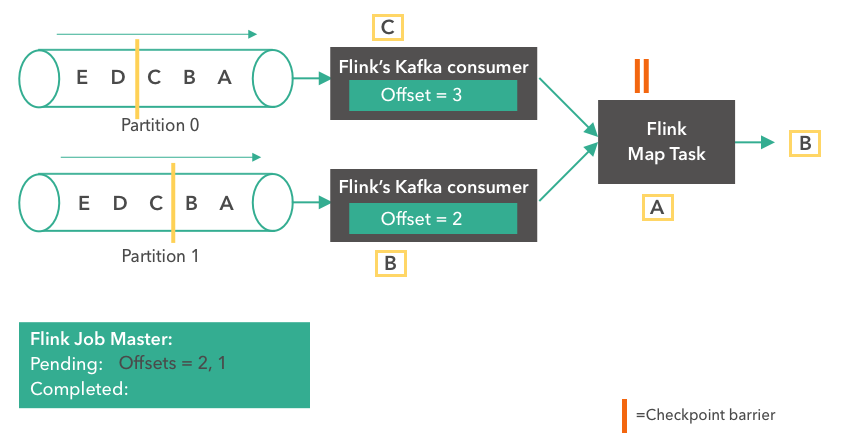浏览(2,548)
•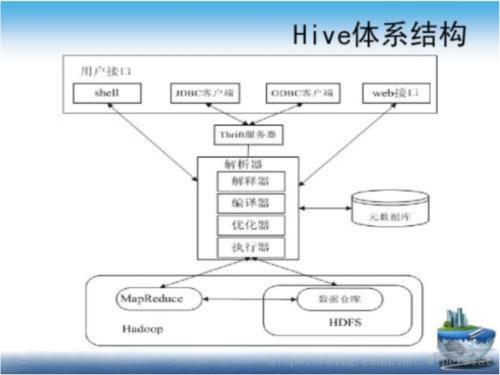浏览(1,776)
•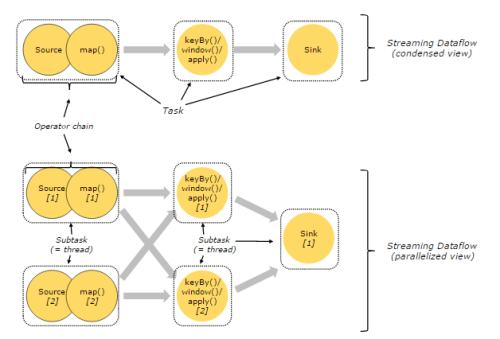浏览(1,739)
•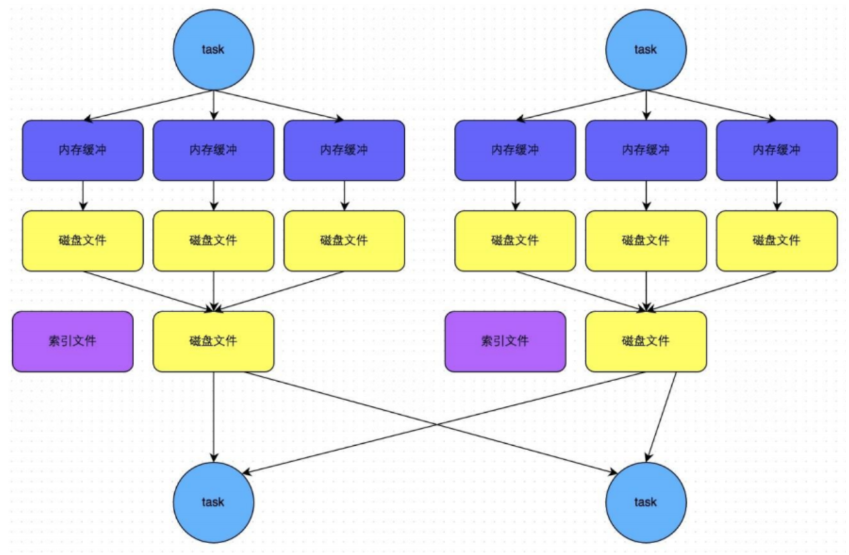浏览(1,732)
•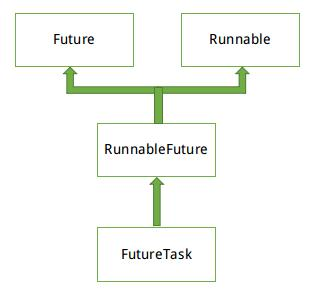浏览(1,685)
•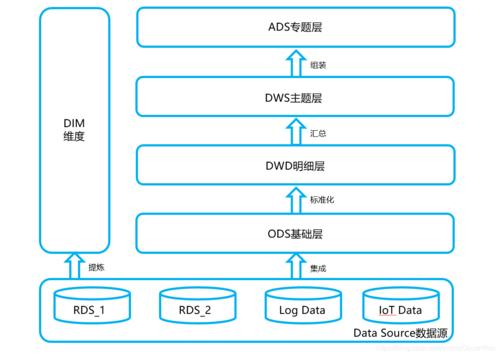浏览(1,673)
•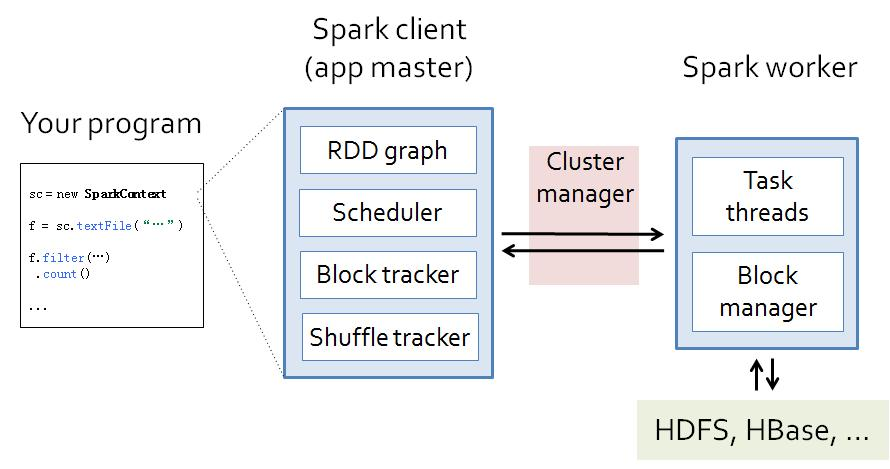浏览(1,640)
•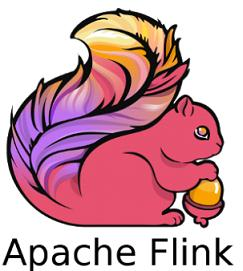浏览(1,613)
•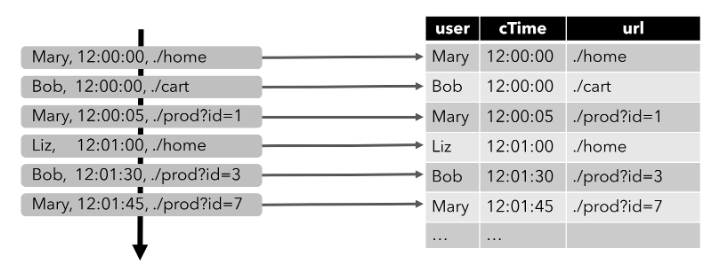浏览(1,537)
•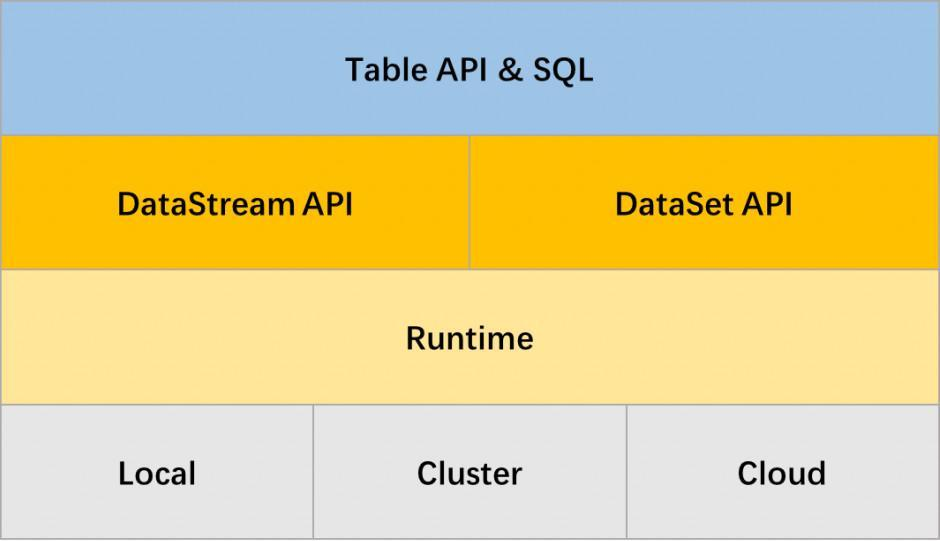浏览(1,474)